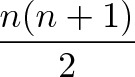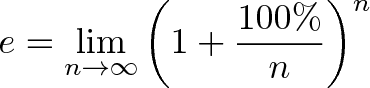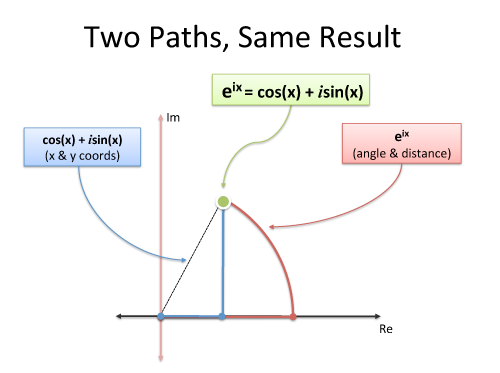- BetterExplained - https://betterexplained.com -

# Math As Language: Understanding the Equals Sign

It’s easy to forget math is a language for communicating ideas. As words, “two and three is equal to five” is cumbersome. Replacing numbers and operations with symbols helps: “2 + 3 is equal to 5”.

But we can do better. In 1557, Robert Recorde invented the equals sign, written with two parallel lines (=), because “noe 2 thynges, can be moare equalle”.

“2 + 3 = 5” is much easier to read. Unfortuantely, the meaning of “equals” changes with the context — just ask programmers who have to distinguish =, == and ===.

A “equals” B is a generic conclusion: what specific relationship are we trying to convey?

Simplification

I see “2 + 3 = 5” as “2 + 3 can be simplified to 5”. The equals sign transitions a complex form on the left to an equivalent, simpler form on the right.

Temporary Assignment

Statements like “speed = 50” mean “the speed is 50, for this scenario”. It’s only good for the problem at hand, and there’s no need to remember this “fact”.

Fundamental Connection

Consider a mathematical truth like $a^2 + b^2 = c^2$, where a, b, and c are the sides of a right triangle.

I read this equals sign as “must always be equal to” or “can be seen as” because it states a permanent relationship, not a coincidence. The arithmetic of $3^2 + 4^2 = 5^2$ is a simplification; the geometry of $a^2 + b^2 = c^2$ is a deep mathematical truth.

The formula to add 1 to n is:$\displaystyle{\frac{n(n+1)}{2}}$

which can be seen as a type of geometric rearrangement, combinatorics, averaging, or even list-making.

Factual Definition

Statements like$\displaystyle{e = \lim_{n\to\infty} \left( 1 + \frac{100\%}{n} \right)^n}$

are definitions of our choosing; the left hand side is a shortcut for the right hand side. It’s similar to temporary assignment, but reserved for “facts” that won’t change between scenarios (e always has the same value in every equation, but “speed” can change).

Constraints

Here’s a tricky one. We might write

x + y = 5

x – y = 3

which indicates conditions we want to be true. I read this as “x + y should be 5, if possible” and “x – y should be 3, if possible”. If we satisfy the constraints (x=4, y=1), great!

If we can’t meet both goals (x + y = 5; 2x + 2y = 9) then the equations could be true individually but not together.

## Example: Demystifying Euler’s Formula

Untangling the equals sign helped me decode Euler’s formula:$\displaystyle{e^{i \cdot \pi} = -1}$

A strange beast, indeed. What type of “equals” is it?

A pedant might say it’s just a simplification and break out the calulus to show it. This isn’t enlightening: there’s a fundamental relationship to discover.

e^i*pi refers to the same destination as -1. Two fingers pointing at the same moon.

They are both ways to describe “the other side of the unit circle, 180 degrees away”. -1 walks there, trodding straight through the grass, while e^i*pi takes the scenic route and rotates through the imaginary dimension. This works for any point on the circle: rotate there, or move in straight lines.Two paths with the same destination: that’s what their equality means. Move beyond a generic equals and find the deeper, specific connection (“simplifies to”, “has been chosen to be”, “refers to the same concept as”).

Happy math.